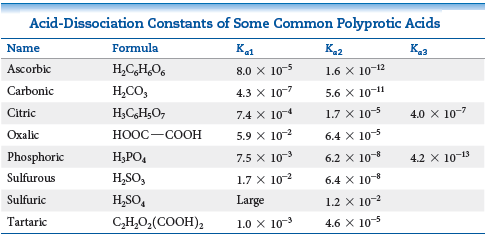# Problem: Using the table below, how many of the following salts are expected to produce acidic solutions: NaHSO4, NaHC2O4, NaH2PO4, and NaHCO3?

###### FREE Expert Solution

Given: NaHSO4, NaHC2O4, NaH2PO4, and NaHCO3

The cation in all of the given salts is Na+: Na+ is a main group metal cation with a +1 charge  Na+ is a neutral ion

Therefore, we have to look at the anion.  Compare Ka and Kb:

Recall that

$\overline{){{\mathbf{K}}}_{{\mathbf{w}}}{\mathbf{=}}{{\mathbf{K}}}_{{\mathbf{a}}}{\mathbf{×}}{{\mathbf{K}}}_{{\mathbf{b}}}}$

The Kb of S2 is:

$\overline{){{\mathbf{K}}}_{{\mathbf{b}}}{\mathbf{=}}\frac{{\mathbf{K}}_{\mathbf{w}}}{{\mathbf{K}}_{\mathbf{a}\mathbf{2}}}}$

For NaHSO4: NaHSO4 dissociates to form Na+ and HSO4.

Anion: HSO4

Ka2 of H2SO4 = 1.2 × 10–2

The Kb of HSO4 is:

${\mathbf{K}}_{\mathbf{b}}\mathbf{=}\frac{\mathbf{1}\mathbf{.}\mathbf{0}\mathbf{×}{\mathbf{10}}^{\mathbf{-}\mathbf{14}}}{\mathbf{1}\mathbf{.}\mathbf{2}\mathbf{×}{\mathbf{10}}^{\mathbf{-}\mathbf{2}}}$

Kb = 8.33 × 10–2

Ka Kb

NaHSO4 will produce an acidic solution.

For NaHC2O4: NaHC2O4 dissociates to form Na+ and HC2O4.

Anion: HC2O4

Ka2 of H2C2O4 = 6.4 × 10–5

92% (468 ratings)###### Problem Details

Using the table below, how many of the following salts are expected to produce acidic solutions: NaHSO4, NaHC2O4, NaH2PO4, and NaHCO3?Frequently Asked Questions

What scientific concept do you need to know in order to solve this problem?

Our tutors have indicated that to solve this problem you will need to apply the Ionic Salts concept. You can view video lessons to learn Ionic Salts. Or if you need more Ionic Salts practice, you can also practice Ionic Salts practice problems.

What professor is this problem relevant for?

Based on our data, we think this problem is relevant for Professor Wade's class at OSU.